Courses

# Test: Machine Design - 1

## 25 Questions MCQ Test Mechanical Engineering SSC JE (Technical) | Test: Machine Design - 1

Description
This mock test of Test: Machine Design - 1 for Mechanical Engineering helps you for every Mechanical Engineering entrance exam. This contains 25 Multiple Choice Questions for Mechanical Engineering Test: Machine Design - 1 (mcq) to study with solutions a complete question bank. The solved questions answers in this Test: Machine Design - 1 quiz give you a good mix of easy questions and tough questions. Mechanical Engineering students definitely take this Test: Machine Design - 1 exercise for a better result in the exam. You can find other Test: Machine Design - 1 extra questions, long questions & short questions for Mechanical Engineering on EduRev as well by searching above.
QUESTION: 1

Solution:
QUESTION: 2

Solution:
QUESTION: 3

### For bolts of uniform strength, the shank diameter is made equal to

Solution:
QUESTION: 4

Match List-I (Part to be Joined) with List-II (Type of Joint) and select the correct answer using the code given below the lists: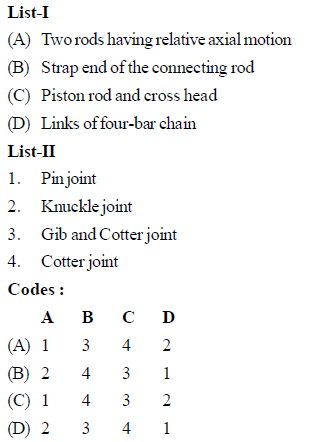Solution:
QUESTION: 5

Match List-I (Items in joints) with List-II (Type of failure) and select the correct answer using the code given below the lists: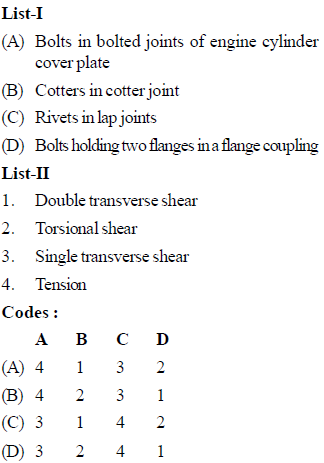Solution:
QUESTION: 6

How can shock absorbing capacity of a bolt be increased

Solution:
QUESTION: 7

Rivets undergo single shear in

Solution:
QUESTION: 8

Which one of the following loadings is considered for design of axles ?

Solution:
QUESTION: 9

Match List-I with List-II and select the correct answer using the codes given below the lists: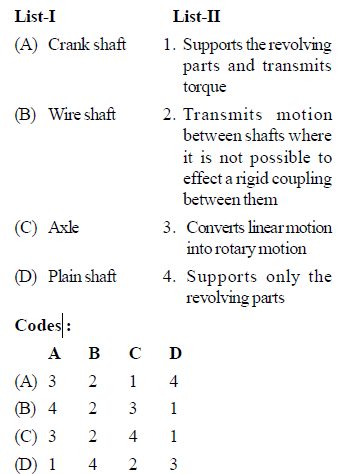Solution:
QUESTION: 10

Removal of metal participles from the raceway of a rolling contact bearing is a kind of failure of bearing known as

Solution:
QUESTION: 11

Match List-I with List-II and select the correct answer using the code given below the lists: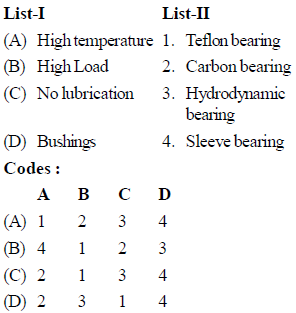Solution:
QUESTION: 12

Match List-I (Machine element) with List-II (Cause of failure) and select the correct answer using the code given below the lists: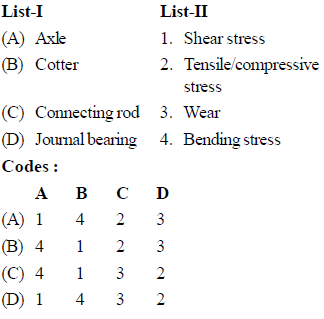Solution:
QUESTION: 13

A transmission shaft subjected to bending loads must be designed on the basis of

Solution:

Explanation : Bending force is normal force, so that design based on maximum normal stress theory.

QUESTION: 14

Tapered roller bearings can take

Solution:
QUESTION: 15

The bearing characteristic number in a hydrodynamic bearing depends on

Solution:
QUESTION: 16

The bearing characteristic number in a hydrodynamic bearing depends upon

Solution:
QUESTION: 17

When a shaft transmits power through gears, the shaft experiences

Solution:

Explanation : Bending stress due to fixing is constant but torsional stresses are varying since speed of shaft varies with the gear speed which is variable

QUESTION: 18

Match List-I with List-II and select the correct answer using the code given below the lists: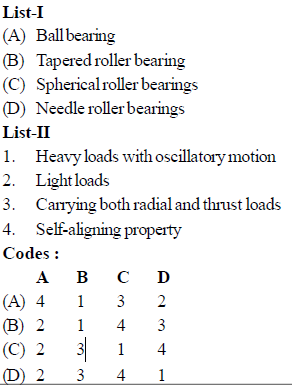Solution:
QUESTION: 19

In a journal bearings, the radius of the friction circle increases with the increase in

Solution:

Radius of friction circle = μ×r

Where

μ= co efficient of friction

QUESTION: 20

In the assembly design of shaft, pulley and ke, the weakest member is

Solution:
QUESTION: 21

In a single row deep groove ball bearing, cages are needed to

Solution:
QUESTION: 22

Solution:
QUESTION: 23

The ratio of the ultimate stress to the design stress is known as

Solution:
QUESTION: 24

The thickness of cotter is generally taken equal to

Solution:
QUESTION: 25

For a cotter, the ratio of thickness to width is

Solution: